# Lesson 2: Visualizing Data Using ggplot2

## Segment 1: Introduction

In data analysis more than anything, a picture really is worth a thousand words. When you start analyzing data in R, your first step shouldn't be to run a complex statistical test: first, you should visualize your data in a graph. This lets you understand the basic nature of the data, so that you know what tests you can perform, and where you should focus your analysis. I'm David Robinson, and in this lesson we'll introduce you to ggplot2, a powerful R package that produces data visualizations easily and intuitively. We will assume you are moderately familiar with basic concepts in R, including variables and functions, and with RStudio, the integrated development environment for programming in R.

So, ggplot2 is a third party package: that means it's code that doesn't come built into the language. This means you have to install it.

You can do that with one line of R code here in your interactive terminal, which is:

and hit return. Or you can go to the Tools->Install Packages menu, where here you type "ggplot2" and hit install.

Each time you reopen R, you need to load the library using the `library` function before you use it. So here that's:

Now we're ready to use it. ggplot2 comes with some data available to use as a demonstration: particularly, the "diamonds" dataset, containing information about several attributes of 54000 diamonds. We can access it using the `data` function:

See that we've added "diamonds" to our global environment. Once we've loaded the diamonds dataset, we can view it using `View`:

Here we have a view of it kind of like a spreadsheet. Here we have the carat: that's the weight of the diamond; and the cut, color and clarity: each of these are measuring something about the quality of the diamond in various levels. And then we have other attributes including the price of the diamond. You can find out what each of these mean using the "help" function:

Here we get a description of the diamonds dataset, and the details about each of the columns.

## Segment 2: Introduction to ggplot2

Let's say that we as scientists are interested in understanding the relationship between those attributes. For example, how does weight, in carats, affect the price? Or how does the quality of the color, or of the diamond's clarity, affect the price? These kinds of questions, where we're looking for interesting relationships among attributes using the observations we have, are common, almost universal, across data analysis.

One common visualization for determining the relationship between attributes is a scatter plot, where each diamond will be represented by one point. This is the point where as graphers, we have to make a few decisions. Let's talk about "aesthetics."

An "aesthetic" is a dimension of a graph that we can perceive visually: the simplest example being the x and y axes. When we make a scatterplot, we choose one attribute to assign to the x axis, and one attribute to assign to the y axis.

Other aesthetics we can use in a scatter plot are the color, size, and shape of the points in the graph: each of these aesthetics lets us communicate some dimension of the data, and understand complex relationships between them.

As an example, let's use ggplot2 to create a scatterplot where we put carat, or weight, on the x axis and price, in dollars, on the y axis. So now we make a ggplot2 call. We start with: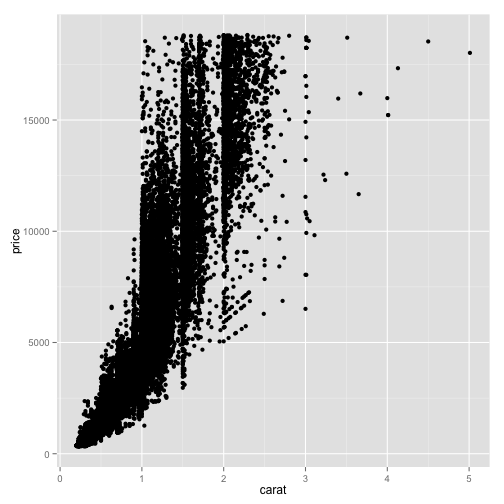Now, there are three parts to a ggplot2 graph. The first is the data we'll be graphing. In this case, we are plotting the diamonds data frame, so we type "diamonds". Second, we show the mapping of aesthetics to the attributes we'll be plotting. We type aes- meaning aesthetics- then open parentheses, and now our assignments: we say "x=carat", saying we want to put carat on the x-axis, then "y=price", saying what we want to put on the y-axis.

Now that we've defined the structure of our graph, we are going to add a "layer" to it: that is, define what time of graph it is. In this case, we want to make a scatter plot: the name for that layer is `geom_point`. "geom" is a typical start for each of these layers. Now we've defined our graph, and hit return, and we see our scatter plot.

See that we've placed "carat" on the x axis, and "price" on the y-axis. Every one of these points represents one row in our data frame: that is, one diamond. We've now communicated a relationship between those two attributes in the dataset: as weight increases, price increases.

Now, this plot shows two aesthetics- weight and price- but there are many other attributes of the data we can communicate. For example, we might want to see how the quality of the cut, or the color, or the clarity, affects the price. Each of those variables is a factor: that means each value belongs to one of a finite number of categories. We can add this using another aesthetic, for example, the color of the points:

To add an aesthetic, we can hit the up arrow to get to our previous line, and then add into the aes call of the aesthetic, "color=clarity", using clarity, which is a measure of the clarity of each diamond, to color our points. We hit return: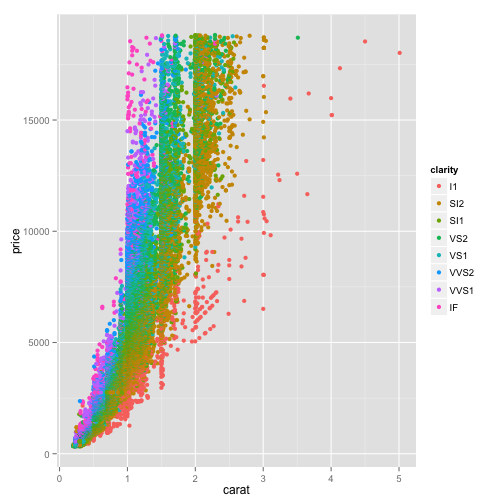Now every point is colored according to the quality of the clarity of each diamond. Notice that it created a legend on the right side. You can see that some of the lighter diamonds are more expensive if they have a high clarity rating, and conversely that some of the heavier diamonds aren't as expensive for having a low clarity rating. This is what leads to this rainbow pattern.

If we would rather see how the quality of the color or cut of the diamond affects the price, we can change the aesthetic. Here in "aes" we change "clarity" to "color".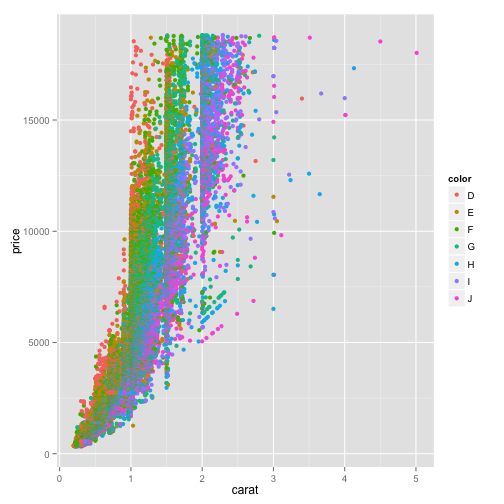Now every item in the color legend is one of the ratings of color. Or we can change it to "cut":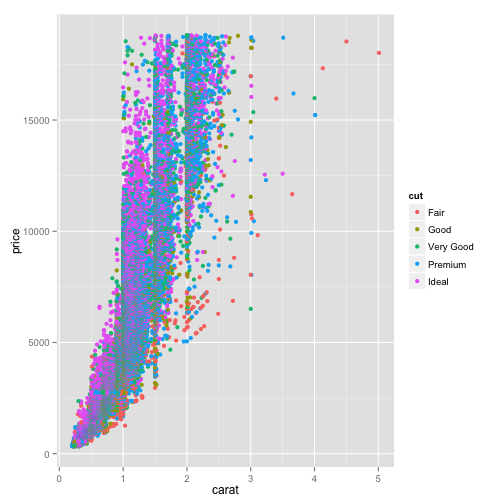This way we can explore the relationship of each of these variables and how it affects the carat/price relationship.

Now, what if we want to see the effect of both color and cut? We can use a fourth aesthetic, such as the size of the points. So here we have color representing the clarity. Let's add another aesthetic- let's say "size=cut."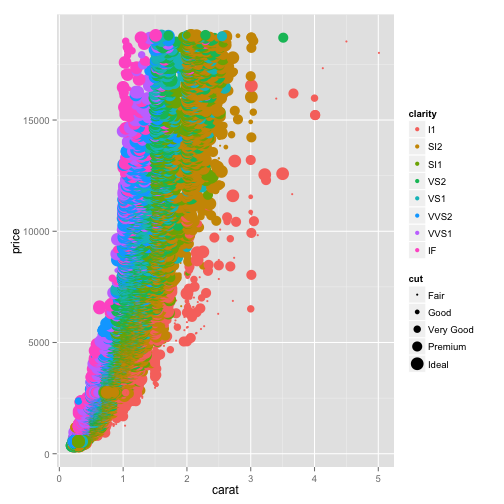Now the size of every point is determined by the cut even while the color is still determined by the clarity. Similarly, we could use the shape to represent the cut: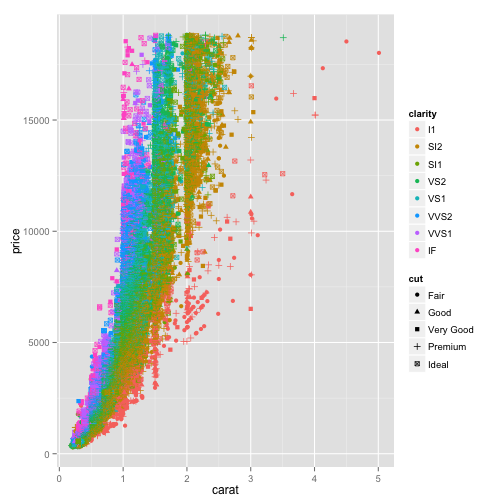Now every shape represents a different cut of the diamond.

Now, this scatter plot is one "layer", which means we can add additional layers besides the scatter plot using the plus sign. For example, what if we want to add a smoothing curve that shows the general trend of the data? That's a layer called `geom_smooth`.

So let's take this plot, take out the color, and add a smoothing trend: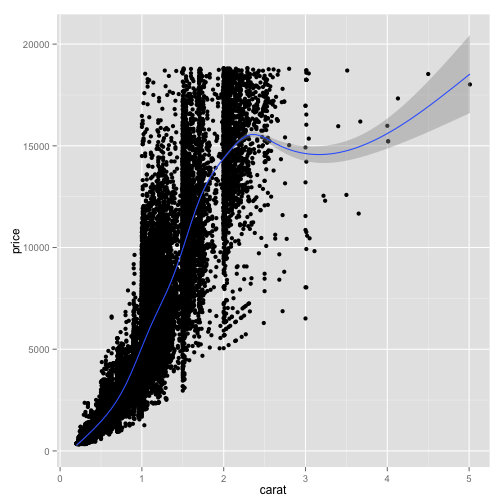The gray area around the curve is a confidence interval, suggesting how much uncertainty there is in this smoothing curve. If we want to turn off the confidence interval, we can add an option to the `geom_smooth` later; specifically "se=FALSE", where "s.e." stands for "standard error."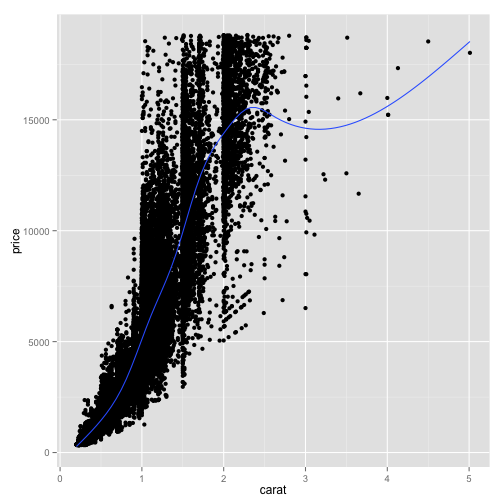This gets rid of the gray area and now we can just see the smoothing curve.

Similarly, if we would rather show a best fit straight line rather than a curve, we can change the "method" option in the `geom_smooth` layer. In this case it's method="lm", where "lm" stands for "Linear model".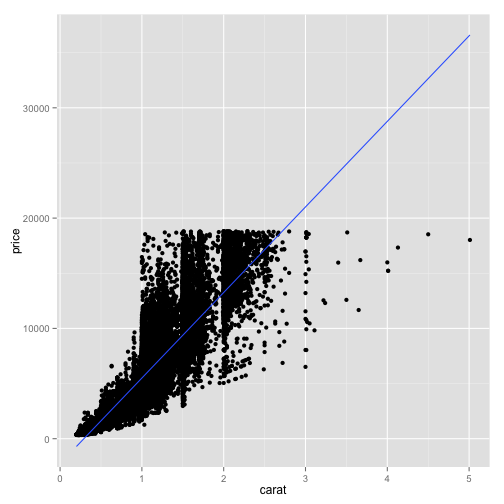There we fit a best fit line to the relationship between carat and price with this `geom_smooth` layer.

If you used a color aesthetic, ggplot will create one smoothing curve for each color. For example, if we add "color=clarity":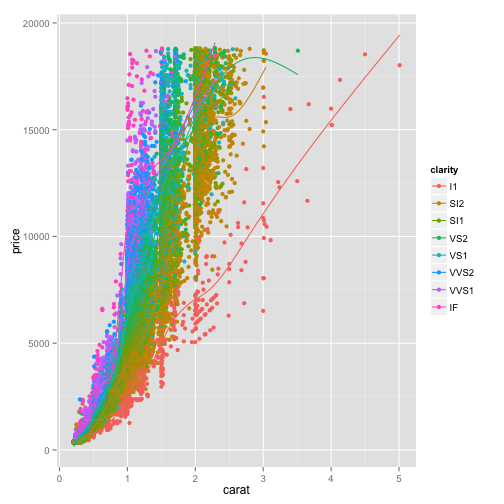Now we see it actually fits one curve for each of these colors. This is a useful way to compare and contrast multiple trends. Note that you can show this smoothing curve layer without showing your scatter plot layer, simply by removing the `geom_point()` layer: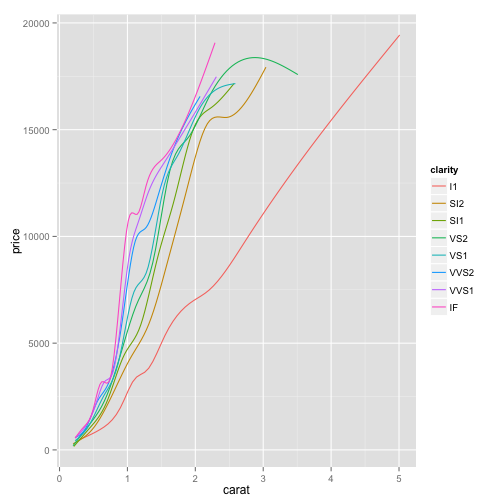This might be a bit clearer: we can see just the fit curves without seeing the actual points.

## Segment 3: Faceting and Additional Options

Another way that you can communicate information about an attribute in your data is to divide your plot up into multiple plots, one for each level, letting you view them separately. This is called "faceting", and ggplot makes it very easy with the "facet_wrap" function.

To do that, we go to a plot like this, and add "facet_wrap(". Now here we put a tilde (~), and then the attribute we would like to divide the plots by, here "clarity."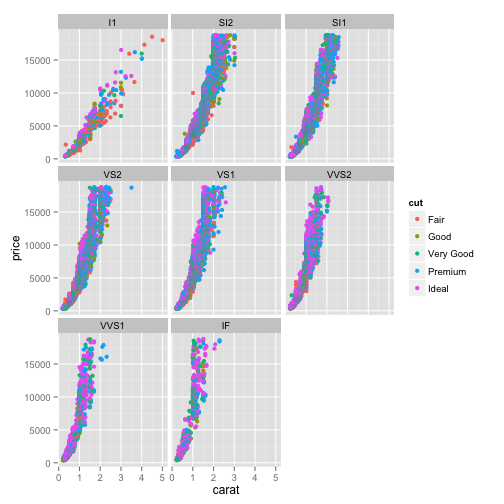Now let's zoom in on this. You can see that now we've divided it into eight subplots, each of which has a different "clarity" value, and you can see how the trend differs between each of those subplots. We can still see that the color is representing the quality of the cut of the diamond.

You can even divide your graph based on two different attributes, such as both color and clarity, using facetgrid. In this case that would be "facetgrid(", then you put "color ~ clarity", where the tilde (~) means "explained by."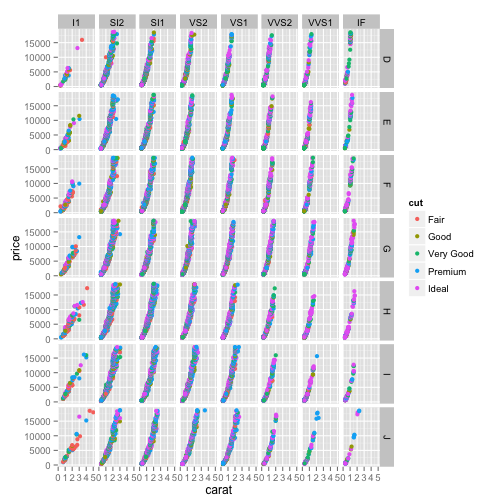Now you can see that each column represents one of the clarity ratings, and each row represents one of the color ratings, and within the combination you can see only those that match that color and that clarity. Faceting like this gives another way to communicate the relationships within your data.

There are many other ways to customize a plot. For starters, you might want to set a title, or set the x or y axis labels manually. You change these options by adding to the end of the line of code. To set the title, you would use the ggtitle function: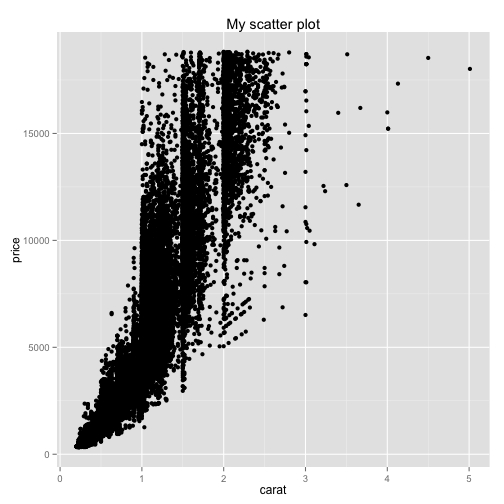This adds a title to the top of your graph. If you'd like to change the x- or y- axis labels, you would add "xlab" for "x label", then your custom label: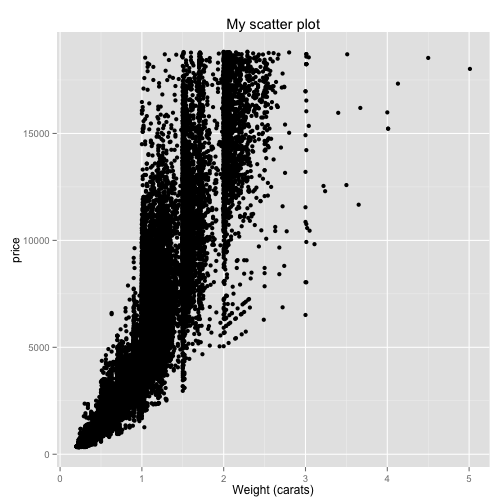You might also want to limit the range of the x or the y axes. You can do this with the xlim or ylim options, which are also added to the end of the line. In this case, say we only want to look at the weights from 0 to 2 carats. We would do: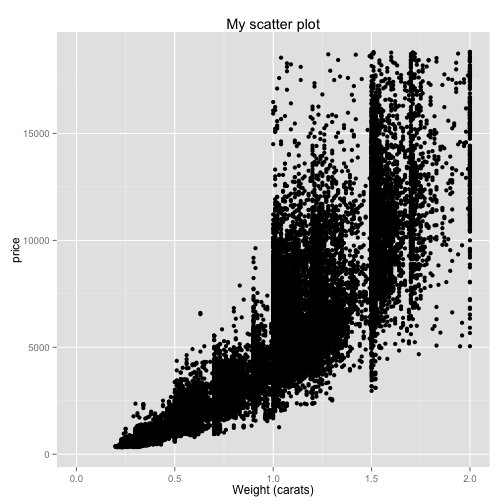Each of these options gets added on after the last one. Now we can see that the x-axis ranges only from 0 to 2. Similarly, if we wanted to show only the y-axis from 0 to 10000, we could put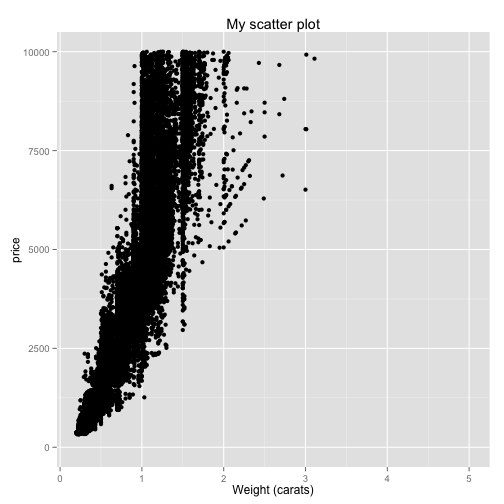Another possibility is to put one of the axes on a log scale. You can do this with the `scale_y_log10()` function.Now you've put the y-axis on a log scale.

There are many other available options and customizations: each gets added to the end of the plot just like these.

## Segment 4: Histograms and Density Plots

We've seen a lot of ways to customize scatter plots. But scatter plots are just one kind of graph. Sometimes we want to look at just one dimension of our data and observe its distribution: for that, we'll use a histogram.

All you need to do to make a histogram is to change your layer from `geom_point()` to `geom_histogram()`. For example, we do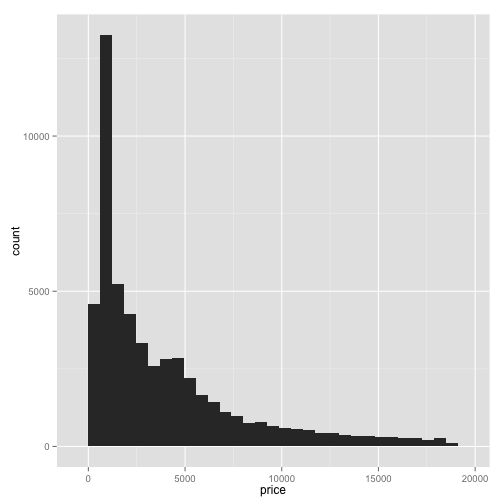This creates a histogram. Notice that we've on the x-axis we've placed price. On the y-axis is the frequency within each bin. This is a visualization of the density of the distribution of price.

You can change the width of each bin as an option to the `geom_histogram` layer. You can make them wider: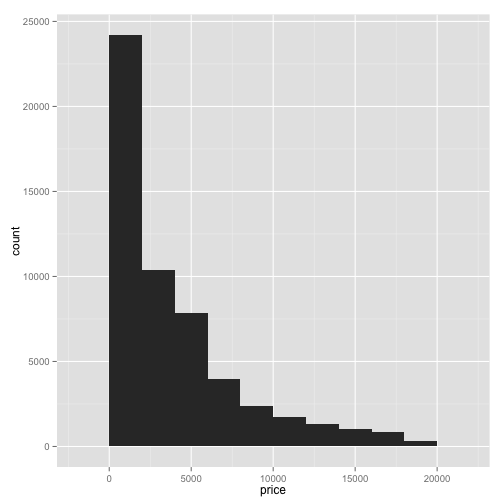Or you can change it to be thinner: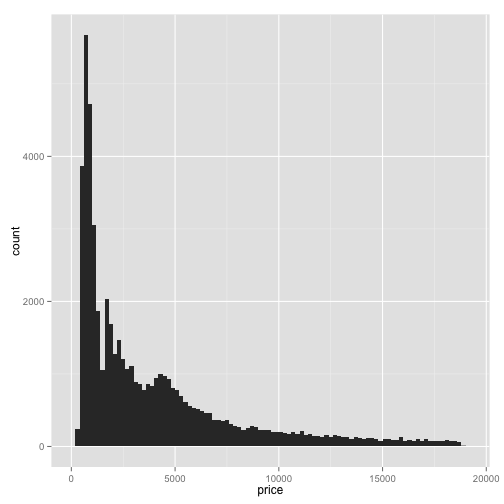Other than that, you can do most of the same things with a histogram that you could with a scatter plot. You can again facet histograms into multiple subplots using facetwrap. For instance, take a plot and use `facetwrap`, and let's divide it by`clarity`: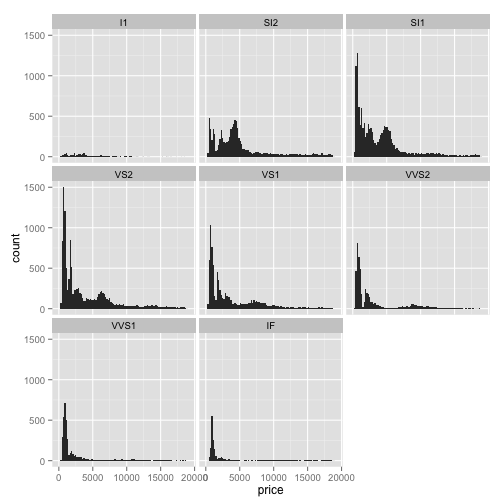Notice that we've created 8 subplots, one for each level of clarity. Note that each subplot shares the same y axis, which might make it hard to interpret the frequencies: some subplots have far more points than others. So to free up the y axis so they can be different between the graphs, we add an argument to facetwrap. In this case, we add `scale="freey"`: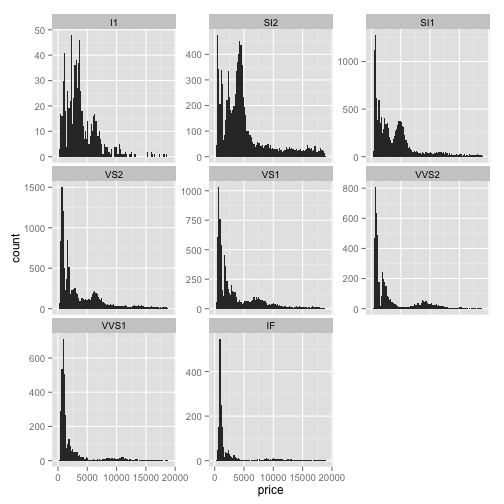Notice that each of the subplots now has a different y-axis; some of them going up to 50, some up to 1000, depending on what is appropriate for that subplot.

Let's say you want to add another attribute to this histogram to see its effect on the density: for example, to make a stacked histogram based on the clarity attribute. Try adding the "fill" aesthetic: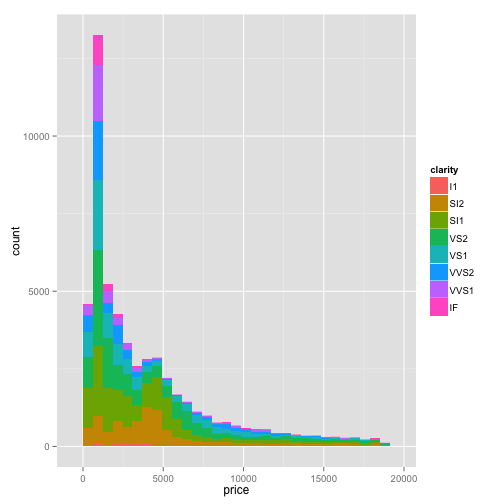This creates a stacked histogram where each color represents the distribution of a different clarity attribute.

You could set this to any other attribute as well, for example the cut: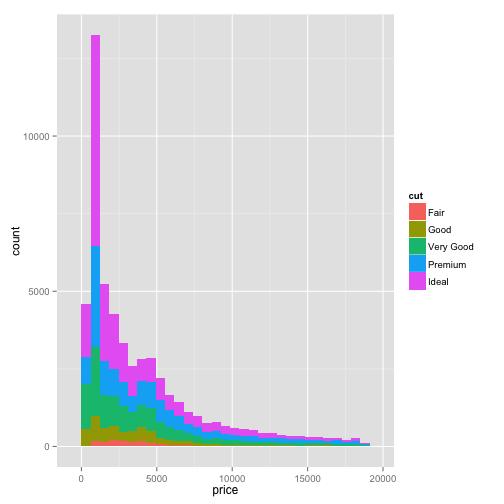Now you can see how the distribution is composed within each of those variables.

Another way to view the distribution is as a density curve. You can do this by changing `geom_histogram` to `geom_density`. Remove the `fill` attribute.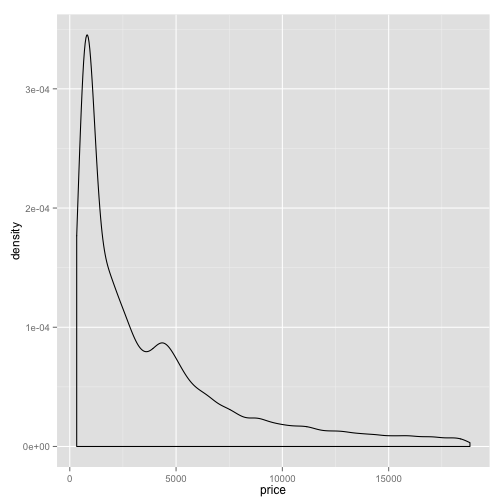Notice it looks smoother than a histogram. If you want to divide this density curve up based on one of your attributes, you can use the `color` aesthetic instead of `fill`. For example, you can add `color=cut`.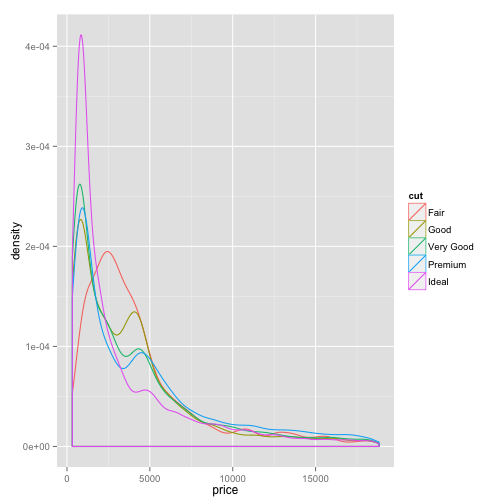This provides a good way to compare multiple distributions.

## Segment 5: Boxplots and Violin Plots

One common method in statistics for comparing multiple densities is to use a boxplot. A boxplot has two attributes: an x, which is usually a classification into categories, and y, the actual variable that you're comparing.

In this case, let's say that you want to compare the distribution of the price within each color. You would do: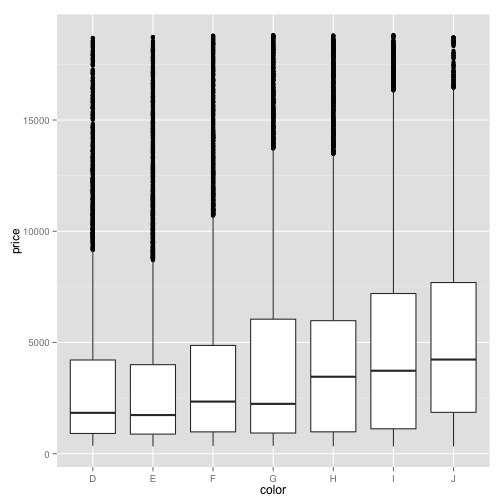The boxplot provides some information in a compact form: you can see the median as a thick black line, the edges of the box show the 25th and 75th quantiles of the data respectively, and these points are outliers that lie far outside the expected range of the data. In this particular case, since there are a large number of outliers you might want to try putting the y-axis on a log scale. Recall that we do that by adding an option: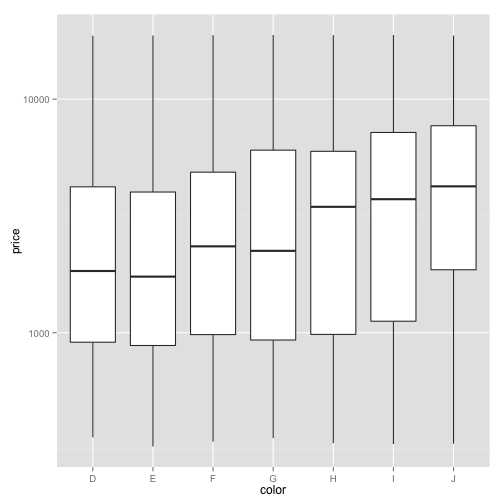This is a better behaved boxplot that gets a better sense of how the distribution of price differs across multiple colors.

One problem with the boxplot is that it doesn't show details of the distribution besides these quantiles. This works well when the data follows a Normal distribution, or a "bell curve," but it might not work well for stranger distributions. For example, the distribution might have not one but two frequency peaks, what we call "bimodality." However strange the distribution, a box plot will always look like a square. We can instead view the distribution as a density using what's called a "violin plot". To do that, all we do is change `geom_boxplot` to `geom_violin`.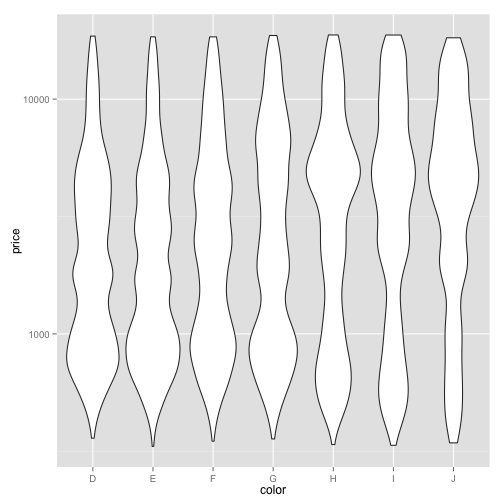The width at each point in this violin plot represents the frequency of that price. So these bumps show the prices that are more common, and we can see that indeed within some colors there is bimodality- there are multiple points that are common- that a boxplot did not represent.

Just like in scatter plots or histograms, if we want to see whether another variable is involved, we can use `facet_wrap` to divide our plot into multiple subplots. For example, we could divide this into subgroups based on clarity. To do that, we would do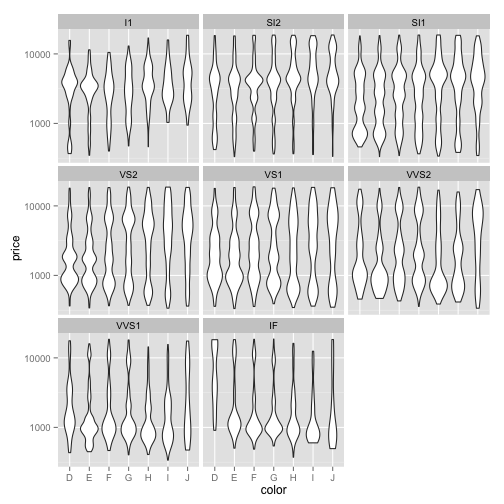Now you can see that we've divided it into eight smaller violin plots showing the distribution within each of those levels of clarity.

## Segment 6: Input- Getting Data into the Right Format

So far all of our analyses have started with a data frame: one row per observation, one column for each attribute. But let's say you have just one vector of numbers and you want to create a histogram, or you have two vectors and want to make a scatterplot. It may not be worth it to construct a data frame with those values just so you can graph it. To make your life easier, ggplot2 provides a simple way to plot one or two vectors, which is the `qplot` function.

Let's generate some random numbers we want to plot. The rnorm function generates random values from a normal distribution, or "bell curve." So if we create a variable `x` with `rnorm`, saying how many random values we want, for example `1000`:

We have created a variable containing 1000 values. Here we can see:

Each of these values was generated from a normal distribution.

Let's say we want to histogram those values. We can give them to the function `qplot`: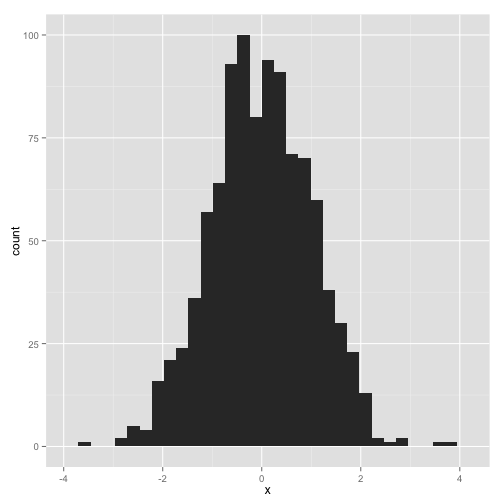This creates a histogram without having to create a data frame or specify `geom_histogram`.

This shortcut also lets us set options easily. For example, we can change the binwidth of the histogram by giving the binwidth argument to qplot: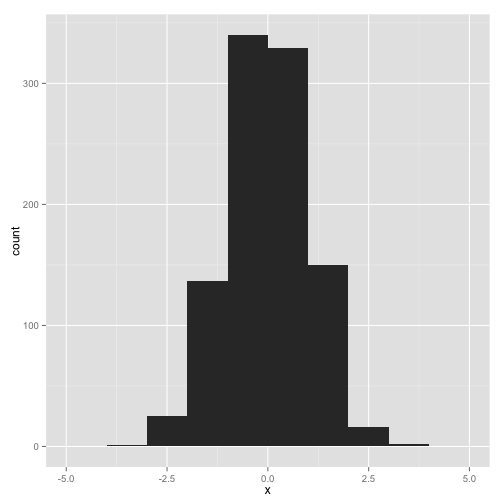We can also set the x and y axis labels easily. We can do that either by adding the `xlab` and `ylab` options like we did before: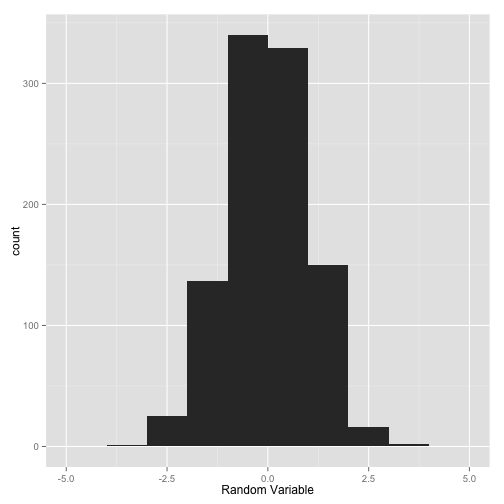Or we can add the `xlab` argument to the `qplot` function.Histograms aren't the only thing we can plot with qplot. Let's create a y variable so that we can construct a scatter plot comparing x to y. Let's make y also be a random normal distribution:

If we want to scatterplot x vs why, we can simply give them to `qplot`.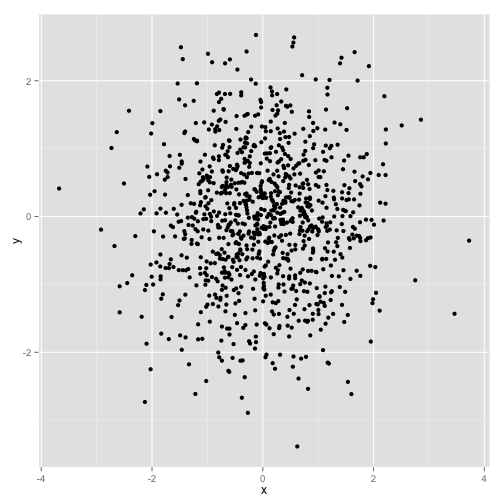Note that we can still add layers to this basic plot just like we could to a regular ggplot call. For example, we could add a smoothing curve with the `geom_smooth` layer: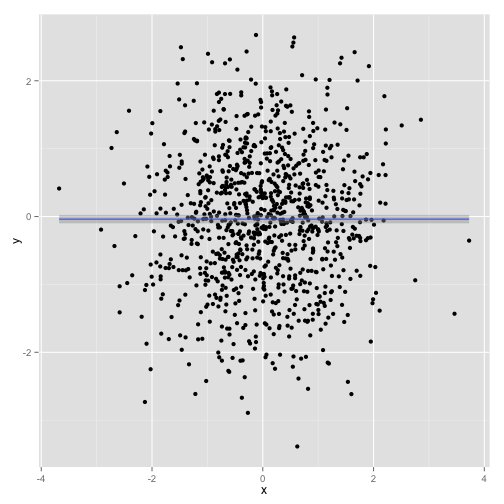This layer ends up on top of the scatterplot created by `qplot`.

Now, so far we've been working with the built-in diamonds dataset. These plots were really easy because the data were given in the format of one observation per row- that is, one diamond per row- which we call "tall" format. But many datasets come in a "wide"" format: that means there is more than one observation- more than one point on your scatterplot- in each row. For example, let's look at the WorldPhones dataset, which comes built into R. Just like we did for diamonds, we use data to load it:

You can see it got added to our global environment. You can then view it using the `View` function.

You can also get more information about WorldPhones using the `help` function:

This dataset shows the number of telephones, measured in thousands, in each continent in each of several years in the 1950s.

Notice that each column is one continent, and each row is one year. Now, that sounds like a reasonable way to store your data. But imagine if we want to compare increases in phone usage between continents, with time on the x axis. That means each point on our plot is going to be one continent in one year. We don't have one observation per row: we have seven! That makes it very difficult to plot using ggplot2.

Luckily, there is an easy way to turn this into tall format, called "melting" the data. To do this, we'll have to install another third party package, called reshape2. Recall that you can do this with

just like we did with `ggplot2`. Now we load the reshape2 package, with

Now we can melt our dataset: that is, turn it from this wide format- many observations per row- to a tall format- one observation per row. Let's assign the new, melted data to a variable called WorldPhones.m, where m is for melted. We assign this using the `melt` function on the WorldPhones data.

Now let's view our new, melted data.

Notice that there are now only three columns: Var1, Var2, and value. So Var1 is year, in Var2 we see each of the continents, and value is the number of phones. What happened was that every cell- every observation- every number of phones per year per continent- in the original data got its own row in this melted data. We can see that in the year 1951, in North America, there were 45 million, 939 thousand phones. You can see the same value in our original unmelted data. So none of the data changed, it just got "reshaped".

To make the data a little more intuitive, you can change the column names. You might recall that we can do this as follows:

So now if we view `WorldPhones.m`, we can see our new column names.

Now that we have our data in melted format, it is easy to create a plot with ggplot2. We go through our usual steps of a ggplot call, but this time we give it WorldPhones.m. We do: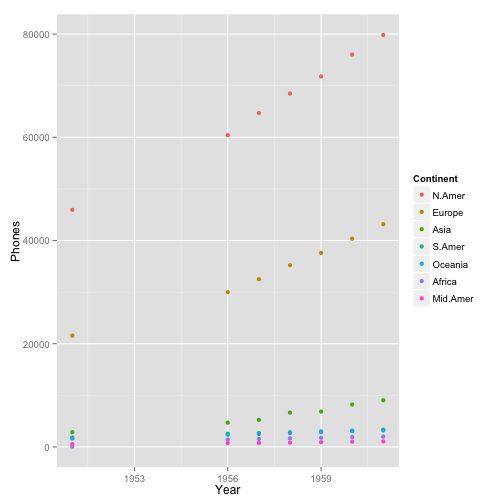on the x-axis we're going to put the Year, so we can see how things change over time. On the y-axis we want to put the number of Phones, which is the variable we're interested in graphing. And let's color each point based on the factor of Continent. Then we'll add the `geom_point` layer to make this into a scatterplot.

Now we've worked with scatterplots before, but since this time what we're showing is a trend over time, it might be better to draw lines between the points in continent. This is easy: we can just change the layer to a `geom_line` layer.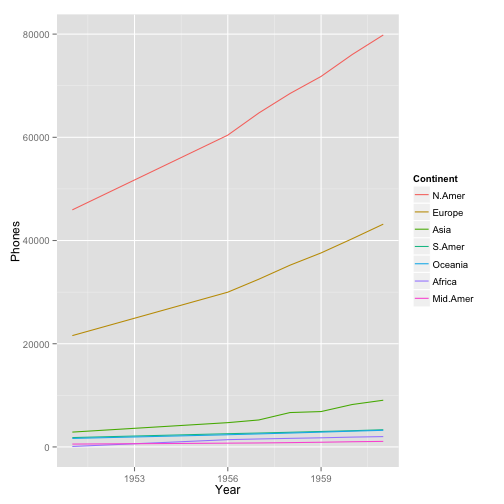Now we have a plot of the number of phones in each continent by year. Incidentally, one might expect phone service to increase exponentially rather than linearly. Also, a lot of the values here are scrunched in the bottom of the axis. When that's the case, it's a good idea to put the y axis on a log scale. Recall that we can do that by adding `scale_y_log10()`.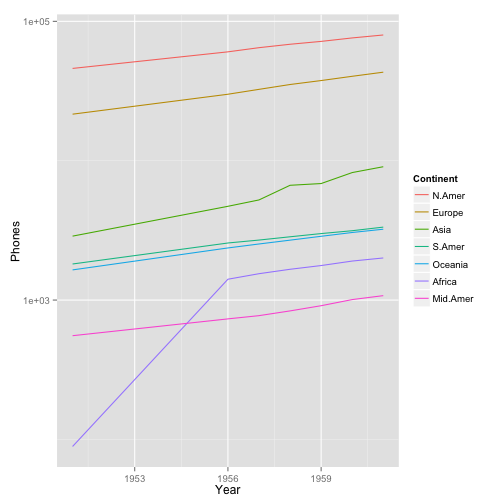Now each of the phone trends looks linear, and we can see the lower values more clearly: for example, that Africa overtook Central America in the number of phones in the year 1956.

Notice how easy this plot was to make once we had the data in the correct format: one row for every point- that's every combination of year and continent- on our graph. So if your data's not in that format when you're starting out, melt it.

## Segment 7: Output: Saving Your Plots

So you just created a great ggplot, and now you want to save it to a file, so you can email it, or add it to your paper or poster, or just look at it later. You can do this with the ggsave function.

First, let's create a scatterplot based on our diamonds data.Now instead of displaying it, let's run that line again, but save it to a variable, called p.

Note that when that happened, the plot did not get recreated: it WAS built but never displayed. We've saved the entire plot into this `p` object. Now we can save that plot to a file, instead of displaying it in our window, by using ggsave:

What just happened is that we created a file called diamonds.png that saved the image. It doesn't have to be a PNG; it can also be a PDF:

or a JPEG:

You can read about the formats ggsave supports, and about the other options you can set like figure height and width, by doing `help` on `ggsave`:

One useful shortcut is that if you just displayed a plot, like in a line like this:Then ggsave will know to save that plot by default when you perform ggsave- you don't even have to tell it which plot you're saving.

Just make sure you're saving the plot you mean to save.

Within RStudio, there's one other choice for saving a plot: you can click on Export, and then "Save Plot As Image," and then select your width, height, filename, and so on.